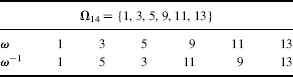## 11.4 TRAP-DOOR KNAPSACKS

In their important paper. Merkle and Hellman  published the first example of a trap-door public-key cryptosystem. They define a transformation relating

• A knapsack problem K{s, t} with a knapsack vector s that is super-increasing and
• A knapsack problem K{a, b, m} modulo m with a seemingly general knapsack vector a.

It was intended that the transformation K{s, t} → K{a, b, m} satisfy three properties:

1. K{a, b, m} and K{s, t} are equivalent, meaning they have a common solution;
2. It is computationally infeasible to find a solution to K{a, b, m};
3. It is easy to find a solution to K{s, t}.

We develop their ideas in this section.

Let SUPn[m] be the subset of SUPn that satisfies the size conditionTABLE 11.3 The Knapsack Multipliers for m = 14Letdenote the set of integers, referred to as knapsack multipliers, which are relatively prime to the modulus m. Each ω m has a multiplicative inverse ω−1 m; that is, 1 = ωω−1 (modulo m).

Note that the modulus m is not required to be a prime number.

Example 11.8

Table 11.3 lists the knapsack multipliers from m = 14.

Example 11.9

Table 11.4 lists the knapsack multipliers for m = 13. When ω is relatively prime to ...

Get Computer Security and Cryptography now with the O’Reilly learning platform.

O’Reilly members experience live online training, plus books, videos, and digital content from nearly 200 publishers.